# How to use the MINVERSE Function in Excel

What is Inverse of a matrix? Why do we need to find the inverse of matrix?

For Systems of linear equation:

Here X is matrix which have unknown values. A & B are known Square matrix.

Given : A X= B

So,   X = A-1B

A-1is inverse of matrix A

X matrix can be known to us, if we just multiply the inverse of A matrix with B matrix. A & B must be square matrix for the operation to be performed.

Square matrix is, which have an equal number of rows and columns in the table.

The MINVERSE function returns the inverse matrix of the provided as an array to the function.

Syntax:

{ =MINVERSE (array) }

array : array of values in a square matrix format (rows = columns).

First we will get the inverse of 1 x 1 matrix.

Use the formula:

{ = MINVERSE ( A1 ) }

Note : don’t use the curly braces using the keyboard symbol. Use the Ctrl + Shift + Enter to apply the curly braces as shown below.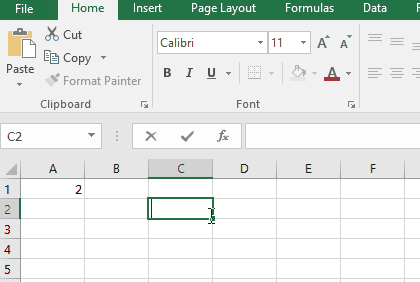The inverse of a 1 x 1 matrix comes out to be 0.5

You can find the inverse of any square matrix
Now we will get the inverse of 5 x 5 matrix.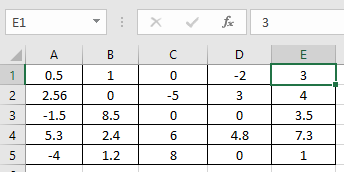Follow the steps to get the inverse of the above given matrix.

1. First select the 25 cells along the table of 5 x 5 dimension as shown below.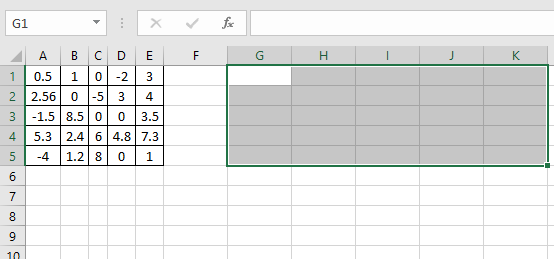2. Now
Use the formula:

{ =MINVERSE ( A1 : E5 ) }

Note : don’t use the curly braces using the keyboard symbol. Use the Ctrl + Shift + Enter to apply the curly braces as shown below.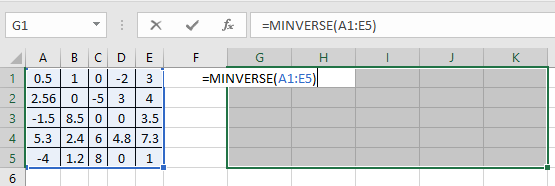3. Now press the Ctrl + Shift + Enter to apply the formula on the selected cell.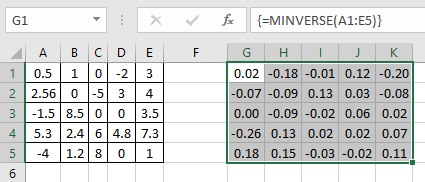Here is it. As you can see here the MINVERSE function returns the matrix of 5 x 5 .

Notes:

1. The function returns the #VALUE! error if there is non numeric value in the array of argument
2. The function returns the #VALUE! error if input array matrix is not a square matrix i.e. rows not equal to columns.
3. The function returns the #NAME? Error is the only value in the array is in non - numeric.
4. Blank cells are not considered as having value zero when calculating the matrix.
5. The function will return values only in the selected cells. If the selected cells are less than the required cells. Then the returned matrix is not the inverse of the given matrix.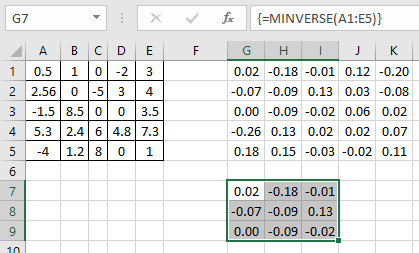6. The function will return values only in the selected cells. If the selected cells are more than the required cells. Then the function returns the inverse of the matrix in the required cells and remaining cells have #NA! error.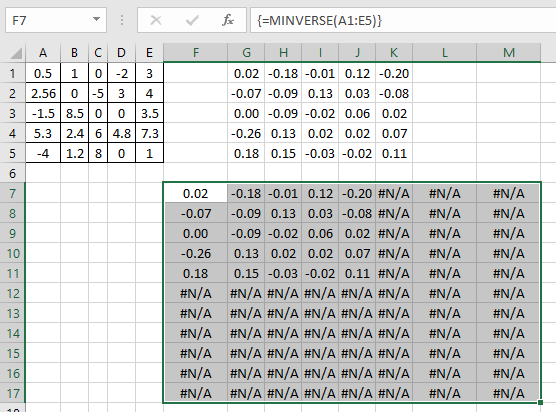7. If you are using Office 365, you wouldn’t have to use the above procedure. You just have to type the formula in one cell =MINVERSE(5) and press Enter to have the inverse matrix 5 x 5 dimension.

Hope you understood how to use MINVERSE function and referring cell in Excel. Explore more articles on Excel mathematical Matrix functions here. Please feel free to state your query or feedback for the above article.

Popular Articles:

50 Excel Shortcuts to Increase Your Productivity

How to use the VLOOKUP Function in Excel

How to use the COUNTIF in Excel 2016

How to use the SUMIF Function in Excel

Terms and Conditions of use

The applications/code on this site are distributed as is and without warranties or liability. In no event shall the owner of the copyrights, or the authors of the applications/code be liable for any loss of profit, any problems or any damage resulting from the use or evaluation of the applications/code.